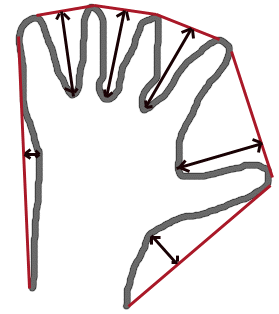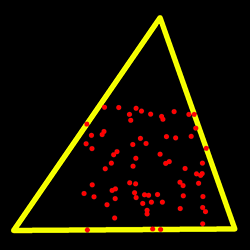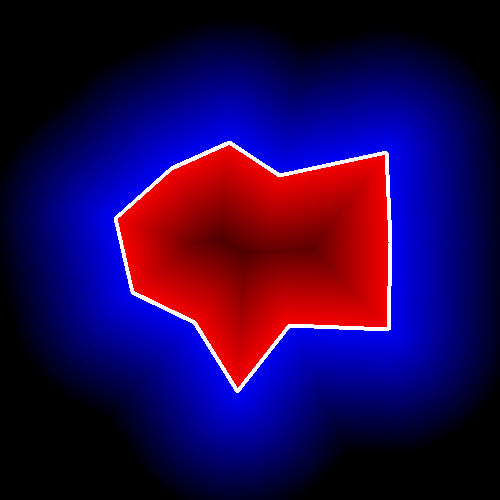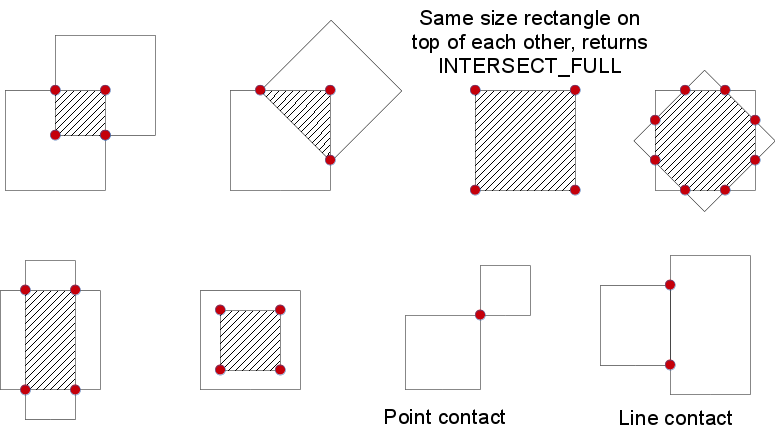OpenCV  3.0.0-rc1 Open Source Computer Vision
Structural Analysis and Shape Descriptors

## Classes

class  cv::Moments
struct returned by cv::moments More...

## Enumerations

enum  cv::ConnectedComponentsTypes {
cv::CC_STAT_LEFT = 0,
cv::CC_STAT_TOP = 1,
cv::CC_STAT_WIDTH = 2,
cv::CC_STAT_HEIGHT = 3,
cv::CC_STAT_AREA = 4,
cv::CC_STAT_MAX = 5
}
connected components algorithm output formats More...

enum  cv::ContourApproximationModes {
cv::CHAIN_APPROX_NONE = 1,
cv::CHAIN_APPROX_SIMPLE = 2,
cv::CHAIN_APPROX_TC89_L1 = 3,
cv::CHAIN_APPROX_TC89_KCOS = 4
}
the contour approximation algorithm More...

enum  cv::RectanglesIntersectTypes {
cv::INTERSECT_NONE = 0,
cv::INTERSECT_PARTIAL = 1,
cv::INTERSECT_FULL = 2
}

enum  cv::RetrievalModes {
cv::RETR_EXTERNAL = 0,
cv::RETR_LIST = 1,
cv::RETR_CCOMP = 2,
cv::RETR_TREE = 3,
cv::RETR_FLOODFILL = 4
}
mode of the contour retrieval algorithm More...

## Functions

void cv::approxPolyDP (InputArray curve, OutputArray approxCurve, double epsilon, bool closed)
Approximates a polygonal curve(s) with the specified precision. More...

double cv::arcLength (InputArray curve, bool closed)
Calculates a contour perimeter or a curve length. More...

Rect cv::boundingRect (InputArray points)
Calculates the up-right bounding rectangle of a point set. More...

void cv::boxPoints (RotatedRect box, OutputArray points)
Finds the four vertices of a rotated rect. Useful to draw the rotated rectangle. More...

int cv::connectedComponents (InputArray image, OutputArray labels, int connectivity=8, int ltype=CV_32S)
computes the connected components labeled image of boolean image More...

int cv::connectedComponentsWithStats (InputArray image, OutputArray labels, OutputArray stats, OutputArray centroids, int connectivity=8, int ltype=CV_32S)

double cv::contourArea (InputArray contour, bool oriented=false)
Calculates a contour area. More...

void cv::convexHull (InputArray points, OutputArray hull, bool clockwise=false, bool returnPoints=true)
Finds the convex hull of a point set. More...

void cv::convexityDefects (InputArray contour, InputArray convexhull, OutputArray convexityDefects)
Finds the convexity defects of a contour. More...

void cv::findContours (InputOutputArray image, OutputArrayOfArrays contours, OutputArray hierarchy, int mode, int method, Point offset=Point())
Finds contours in a binary image. More...

void cv::findContours (InputOutputArray image, OutputArrayOfArrays contours, int mode, int method, Point offset=Point())

RotatedRect cv::fitEllipse (InputArray points)
Fits an ellipse around a set of 2D points. More...

void cv::fitLine (InputArray points, OutputArray line, int distType, double param, double reps, double aeps)
Fits a line to a 2D or 3D point set. More...

void cv::HuMoments (const Moments &moments, double hu)
Calculates seven Hu invariants. More...

void cv::HuMoments (const Moments &m, OutputArray hu)

float cv::intersectConvexConvex (InputArray _p1, InputArray _p2, OutputArray _p12, bool handleNested=true)
finds intersection of two convex polygons More...

bool cv::isContourConvex (InputArray contour)
Tests a contour convexity. More...

double cv::matchShapes (InputArray contour1, InputArray contour2, int method, double parameter)
Compares two shapes. More...

RotatedRect cv::minAreaRect (InputArray points)
Finds a rotated rectangle of the minimum area enclosing the input 2D point set. More...

void cv::minEnclosingCircle (InputArray points, Point2f &center, float &radius)
Finds a circle of the minimum area enclosing a 2D point set. More...

double cv::minEnclosingTriangle (InputArray points, OutputArray triangle)
Finds a triangle of minimum area enclosing a 2D point set and returns its area. More...

Moments cv::moments (InputArray array, bool binaryImage=false)
Calculates all of the moments up to the third order of a polygon or rasterized shape. More...

double cv::pointPolygonTest (InputArray contour, Point2f pt, bool measureDist)
Performs a point-in-contour test. More...

int cv::rotatedRectangleIntersection (const RotatedRect &rect1, const RotatedRect &rect2, OutputArray intersectingRegion)
Finds out if there is any intersection between two rotated rectangles. More...

## Enumeration Type Documentation

connected components algorithm output formats

Enumerator
CC_STAT_LEFT

The leftmost (x) coordinate which is the inclusive start of the bounding box in the horizontal direction.

CC_STAT_TOP

The topmost (y) coordinate which is the inclusive start of the bounding box in the vertical direction.

CC_STAT_WIDTH

The horizontal size of the bounding box.

CC_STAT_HEIGHT

The vertical size of the bounding box.

CC_STAT_AREA

The total area (in pixels) of the connected component.

CC_STAT_MAX

the contour approximation algorithm

Enumerator
CHAIN_APPROX_NONE

stores absolutely all the contour points. That is, any 2 subsequent points (x1,y1) and (x2,y2) of the contour will be either horizontal, vertical or diagonal neighbors, that is, max(abs(x1-x2),abs(y2-y1))==1.

CHAIN_APPROX_SIMPLE

compresses horizontal, vertical, and diagonal segments and leaves only their end points. For example, an up-right rectangular contour is encoded with 4 points.

CHAIN_APPROX_TC89_L1

applies one of the flavors of the Teh-Chin chain approximation algorithm 

CHAIN_APPROX_TC89_KCOS

applies one of the flavors of the Teh-Chin chain approximation algorithm 

types of intersection between rectangles

Enumerator
INTERSECT_NONE

No intersection.

INTERSECT_PARTIAL

There is a partial intersection.

INTERSECT_FULL

One of the rectangle is fully enclosed in the other.

 enum cv::RetrievalModes

mode of the contour retrieval algorithm

Enumerator
RETR_EXTERNAL

retrieves only the extreme outer contours. It sets hierarchy[i]=hierarchy[i]=-1 for all the contours.

RETR_LIST

retrieves all of the contours without establishing any hierarchical relationships.

RETR_CCOMP

retrieves all of the contours and organizes them into a two-level hierarchy. At the top level, there are external boundaries of the components. At the second level, there are boundaries of the holes. If there is another contour inside a hole of a connected component, it is still put at the top level.

RETR_TREE

retrieves all of the contours and reconstructs a full hierarchy of nested contours.

RETR_FLOODFILL

## Function Documentation

 void cv::approxPolyDP ( InputArray curve, OutputArray approxCurve, double epsilon, bool closed )

Approximates a polygonal curve(s) with the specified precision.

The functions approxPolyDP approximate a curve or a polygon with another curve/polygon with less vertices so that the distance between them is less or equal to the specified precision. It uses the Douglas-Peucker algorithm http://en.wikipedia.org/wiki/Ramer-Douglas-Peucker_algorithm

Parameters
 curve Input vector of a 2D point stored in std::vector or Mat approxCurve Result of the approximation. The type should match the type of the input curve. epsilon Parameter specifying the approximation accuracy. This is the maximum distance between the original curve and its approximation. closed If true, the approximated curve is closed (its first and last vertices are connected). Otherwise, it is not closed.
Examples:
contours2.cpp.
 double cv::arcLength ( InputArray curve, bool closed )

Calculates a contour perimeter or a curve length.

The function computes a curve length or a closed contour perimeter.

Parameters
 curve Input vector of 2D points, stored in std::vector or Mat. closed Flag indicating whether the curve is closed or not.
 Rect cv::boundingRect ( InputArray points )

Calculates the up-right bounding rectangle of a point set.

The function calculates and returns the minimal up-right bounding rectangle for the specified point set.

Parameters
 points Input 2D point set, stored in std::vector or Mat.
 void cv::boxPoints ( RotatedRect box, OutputArray points )

Finds the four vertices of a rotated rect. Useful to draw the rotated rectangle.

The function finds the four vertices of a rotated rectangle. This function is useful to draw the rectangle. In C++, instead of using this function, you can directly use box.points() method. Please visit the tutorial on bounding rectangle for more information.

Parameters
 box The input rotated rectangle. It may be the output of points The output array of four vertices of rectangles.
 int cv::connectedComponents ( InputArray image, OutputArray labels, int connectivity = 8, int ltype = CV_32S )

computes the connected components labeled image of boolean image

image with 4 or 8 way connectivity - returns N, the total number of labels [0, N-1] where 0 represents the background label. ltype specifies the output label image type, an important consideration based on the total number of labels or alternatively the total number of pixels in the source image.

Parameters
 image the image to be labeled labels destination labeled image connectivity 8 or 4 for 8-way or 4-way connectivity respectively ltype output image label type. Currently CV_32S and CV_16U are supported.
 int cv::connectedComponentsWithStats ( InputArray image, OutputArray labels, OutputArray stats, OutputArray centroids, int connectivity = 8, int ltype = CV_32S )

This is an overloaded member function, provided for convenience. It differs from the above function only in what argument(s) it accepts.

Parameters
 image the image to be labeled labels destination labeled image stats statistics output for each label, including the background label, see below for available statistics. Statistics are accessed via stats(label, COLUMN) where COLUMN is one of cv::ConnectedComponentsTypes centroids floating point centroid (x,y) output for each label, including the background label connectivity 8 or 4 for 8-way or 4-way connectivity respectively ltype output image label type. Currently CV_32S and CV_16U are supported.
 double cv::contourArea ( InputArray contour, bool oriented = false )

Calculates a contour area.

The function computes a contour area. Similarly to moments , the area is computed using the Green formula. Thus, the returned area and the number of non-zero pixels, if you draw the contour using drawContours or fillPoly , can be different. Also, the function will most certainly give a wrong results for contours with self-intersections.

Example:

vector<Point> contour;
contour.push_back(Point2f(0, 0));
contour.push_back(Point2f(10, 0));
contour.push_back(Point2f(10, 10));
contour.push_back(Point2f(5, 4));
double area0 = contourArea(contour);
vector<Point> approx;
approxPolyDP(contour, approx, 5, true);
double area1 = contourArea(approx);
cout << "area0 =" << area0 << endl <<
"area1 =" << area1 << endl <<
"approx poly vertices" << approx.size() << endl;
Parameters
 contour Input vector of 2D points (contour vertices), stored in std::vector or Mat. oriented Oriented area flag. If it is true, the function returns a signed area value, depending on the contour orientation (clockwise or counter-clockwise). Using this feature you can determine orientation of a contour by taking the sign of an area. By default, the parameter is false, which means that the absolute value is returned.
Examples:
segment_objects.cpp.
 void cv::convexHull ( InputArray points, OutputArray hull, bool clockwise = false, bool returnPoints = true )

Finds the convex hull of a point set.

The functions find the convex hull of a 2D point set using the Sklansky's algorithm  that has O(N logN) complexity in the current implementation. See the OpenCV sample convexhull.cpp that demonstrates the usage of different function variants.

Parameters
 points Input 2D point set, stored in std::vector or Mat. hull Output convex hull. It is either an integer vector of indices or vector of points. In the first case, the hull elements are 0-based indices of the convex hull points in the original array (since the set of convex hull points is a subset of the original point set). In the second case, hull elements are the convex hull points themselves. clockwise Orientation flag. If it is true, the output convex hull is oriented clockwise. Otherwise, it is oriented counter-clockwise. The assumed coordinate system has its X axis pointing to the right, and its Y axis pointing upwards. returnPoints Operation flag. In case of a matrix, when the flag is true, the function returns convex hull points. Otherwise, it returns indices of the convex hull points. When the output array is std::vector, the flag is ignored, and the output depends on the type of the vector: std::vector implies returnPoints=true, std::vector implies returnPoints=false.
Examples:
convexhull.cpp.
 void cv::convexityDefects ( InputArray contour, InputArray convexhull, OutputArray convexityDefects )

Finds the convexity defects of a contour.

The figure below displays convexity defects of a hand contour:image
Parameters
 contour Input contour. convexhull Convex hull obtained using convexHull that should contain indices of the contour points that make the hull. convexityDefects The output vector of convexity defects. In C++ and the new Python/Java interface each convexity defect is represented as 4-element integer vector (a.k.a. cv::Vec4i): (start_index, end_index, farthest_pt_index, fixpt_depth), where indices are 0-based indices in the original contour of the convexity defect beginning, end and the farthest point, and fixpt_depth is fixed-point approximation (with 8 fractional bits) of the distance between the farthest contour point and the hull. That is, to get the floating-point value of the depth will be fixpt_depth/256.0.
 void cv::findContours ( InputOutputArray image, OutputArrayOfArrays contours, OutputArray hierarchy, int mode, int method, Point offset = Point() )

Finds contours in a binary image.

The function retrieves contours from the binary image using the algorithm  . The contours are a useful tool for shape analysis and object detection and recognition. See squares.c in the OpenCV sample directory.

Note
Source image is modified by this function. Also, the function does not take into account 1-pixel border of the image (it's filled with 0's and used for neighbor analysis in the algorithm), therefore the contours touching the image border will be clipped.
Parameters
 image Source, an 8-bit single-channel image. Non-zero pixels are treated as 1's. Zero pixels remain 0's, so the image is treated as binary . You can use compare , inRange , threshold , adaptiveThreshold , Canny , and others to create a binary image out of a grayscale or color one. The function modifies the image while extracting the contours. If mode equals to RETR_CCOMP or RETR_FLOODFILL, the input can also be a 32-bit integer image of labels (CV_32SC1). contours Detected contours. Each contour is stored as a vector of points. hierarchy Optional output vector, containing information about the image topology. It has as many elements as the number of contours. For each i-th contour contours[i] , the elements hierarchy[i] , hiearchy[i] , hiearchy[i] , and hiearchy[i] are set to 0-based indices in contours of the next and previous contours at the same hierarchical level, the first child contour and the parent contour, respectively. If for the contour i there are no next, previous, parent, or nested contours, the corresponding elements of hierarchy[i] will be negative. mode Contour retrieval mode, see cv::RetrievalModes method Contour approximation method, see cv::ContourApproximationModes offset Optional offset by which every contour point is shifted. This is useful if the contours are extracted from the image ROI and then they should be analyzed in the whole image context.
Examples:
contours2.cpp, fitellipse.cpp, segment_objects.cpp, and watershed.cpp.
 void cv::findContours ( InputOutputArray image, OutputArrayOfArrays contours, int mode, int method, Point offset = Point() )

This is an overloaded member function, provided for convenience. It differs from the above function only in what argument(s) it accepts.

 RotatedRect cv::fitEllipse ( InputArray points )

Fits an ellipse around a set of 2D points.

The function calculates the ellipse that fits (in a least-squares sense) a set of 2D points best of all. It returns the rotated rectangle in which the ellipse is inscribed. The first algorithm described by  is used. Developer should keep in mind that it is possible that the returned ellipse/rotatedRect data contains negative indices, due to the data points being close to the border of the containing Mat element.

Parameters
 points Input 2D point set, stored in std::vector<> or Mat
Examples:
fitellipse.cpp.
 void cv::fitLine ( InputArray points, OutputArray line, int distType, double param, double reps, double aeps )

Fits a line to a 2D or 3D point set.

The function fitLine fits a line to a 2D or 3D point set by minimizing $$\sum_i \rho(r_i)$$ where $$r_i$$ is a distance between the $$i^{th}$$ point, the line and $$\rho(r)$$ is a distance function, one of the following:

• DIST_L2

$\rho (r) = r^2/2 \quad \text{(the simplest and the fastest least-squares method)}$

• DIST_L1

$\rho (r) = r$

• DIST_L12

$\rho (r) = 2 \cdot ( \sqrt{1 + \frac{r^2}{2}} - 1)$

• DIST_FAIR

$\rho \left (r \right ) = C^2 \cdot \left ( \frac{r}{C} - \log{\left(1 + \frac{r}{C}\right)} \right ) \quad \text{where} \quad C=1.3998$

• DIST_WELSCH

$\rho \left (r \right ) = \frac{C^2}{2} \cdot \left ( 1 - \exp{\left(-\left(\frac{r}{C}\right)^2\right)} \right ) \quad \text{where} \quad C=2.9846$

• DIST_HUBER

$\rho (r) = \fork{r^2/2}{if $$r < C$$}{C \cdot (r-C/2)}{otherwise} \quad \text{where} \quad C=1.345$

The algorithm is based on the M-estimator ( http://en.wikipedia.org/wiki/M-estimator ) technique that iteratively fits the line using the weighted least-squares algorithm. After each iteration the weights $$w_i$$ are adjusted to be inversely proportional to $$\rho(r_i)$$ .

Parameters
 points Input vector of 2D or 3D points, stored in std::vector<> or Mat. line Output line parameters. In case of 2D fitting, it should be a vector of 4 elements (like Vec4f) - (vx, vy, x0, y0), where (vx, vy) is a normalized vector collinear to the line and (x0, y0) is a point on the line. In case of 3D fitting, it should be a vector of 6 elements (like Vec6f) - (vx, vy, vz, x0, y0, z0), where (vx, vy, vz) is a normalized vector collinear to the line and (x0, y0, z0) is a point on the line. distType Distance used by the M-estimator, see cv::DistanceTypes param Numerical parameter ( C ) for some types of distances. If it is 0, an optimal value is chosen. reps Sufficient accuracy for the radius (distance between the coordinate origin and the line). aeps Sufficient accuracy for the angle. 0.01 would be a good default value for reps and aeps.
 void cv::HuMoments ( const Moments & moments, double hu )

Calculates seven Hu invariants.

The function calculates seven Hu invariants (introduced in ; see also http://en.wikipedia.org/wiki/Image_moment) defined as:

$\begin{array}{l} hu= \eta _{20}+ \eta _{02} \\ hu=( \eta _{20}- \eta _{02})^{2}+4 \eta _{11}^{2} \\ hu=( \eta _{30}-3 \eta _{12})^{2}+ (3 \eta _{21}- \eta _{03})^{2} \\ hu=( \eta _{30}+ \eta _{12})^{2}+ ( \eta _{21}+ \eta _{03})^{2} \\ hu=( \eta _{30}-3 \eta _{12})( \eta _{30}+ \eta _{12})[( \eta _{30}+ \eta _{12})^{2}-3( \eta _{21}+ \eta _{03})^{2}]+(3 \eta _{21}- \eta _{03})( \eta _{21}+ \eta _{03})[3( \eta _{30}+ \eta _{12})^{2}-( \eta _{21}+ \eta _{03})^{2}] \\ hu=( \eta _{20}- \eta _{02})[( \eta _{30}+ \eta _{12})^{2}- ( \eta _{21}+ \eta _{03})^{2}]+4 \eta _{11}( \eta _{30}+ \eta _{12})( \eta _{21}+ \eta _{03}) \\ hu=(3 \eta _{21}- \eta _{03})( \eta _{21}+ \eta _{03})[3( \eta _{30}+ \eta _{12})^{2}-( \eta _{21}+ \eta _{03})^{2}]-( \eta _{30}-3 \eta _{12})( \eta _{21}+ \eta _{03})[3( \eta _{30}+ \eta _{12})^{2}-( \eta _{21}+ \eta _{03})^{2}] \\ \end{array}$

where $$\eta_{ji}$$ stands for $$\texttt{Moments::nu}_{ji}$$ .

These values are proved to be invariants to the image scale, rotation, and reflection except the seventh one, whose sign is changed by reflection. This invariance is proved with the assumption of infinite image resolution. In case of raster images, the computed Hu invariants for the original and transformed images are a bit different.

Parameters
 moments Input moments computed with moments . hu Output Hu invariants.
matchShapes
 void cv::HuMoments ( const Moments & m, OutputArray hu )

This is an overloaded member function, provided for convenience. It differs from the above function only in what argument(s) it accepts.

 float cv::intersectConvexConvex ( InputArray _p1, InputArray _p2, OutputArray _p12, bool handleNested = true )

finds intersection of two convex polygons

 bool cv::isContourConvex ( InputArray contour )

Tests a contour convexity.

The function tests whether the input contour is convex or not. The contour must be simple, that is, without self-intersections. Otherwise, the function output is undefined.

Parameters
 contour Input vector of 2D points, stored in std::vector<> or Mat
 double cv::matchShapes ( InputArray contour1, InputArray contour2, int method, double parameter )

Compares two shapes.

The function compares two shapes. All three implemented methods use the Hu invariants (see cv::HuMoments)

Parameters
 contour1 First contour or grayscale image. contour2 Second contour or grayscale image. method Comparison method, see ShapeMatchModes parameter Method-specific parameter (not supported now).
 RotatedRect cv::minAreaRect ( InputArray points )

Finds a rotated rectangle of the minimum area enclosing the input 2D point set.

The function calculates and returns the minimum-area bounding rectangle (possibly rotated) for a specified point set. See the OpenCV sample minarea.cpp . Developer should keep in mind that the returned rotatedRect can contain negative indices when data is close the the containing Mat element boundary.

Parameters
 points Input vector of 2D points, stored in std::vector<> or Mat
Examples:
minarea.cpp.
 void cv::minEnclosingCircle ( InputArray points, Point2f & center, float & radius )

Finds a circle of the minimum area enclosing a 2D point set.

The function finds the minimal enclosing circle of a 2D point set using an iterative algorithm. See the OpenCV sample minarea.cpp .

Parameters
 points Input vector of 2D points, stored in std::vector<> or Mat center Output center of the circle. radius Output radius of the circle.
Examples:
minarea.cpp.
 double cv::minEnclosingTriangle ( InputArray points, OutputArray triangle )

Finds a triangle of minimum area enclosing a 2D point set and returns its area.

The function finds a triangle of minimum area enclosing the given set of 2D points and returns its area. The output for a given 2D point set is shown in the image below. 2D points are depicted in red* and the enclosing triangle in yellow.Sample output of the minimum enclosing triangle function

The implementation of the algorithm is based on O'Rourke's  and Klee and Laskowski's  papers. O'Rourke provides a $$\theta(n)$$ algorithm for finding the minimal enclosing triangle of a 2D convex polygon with n vertices. Since the minEnclosingTriangle function takes a 2D point set as input an additional preprocessing step of computing the convex hull of the 2D point set is required. The complexity of the convexHull function is $$O(n log(n))$$ which is higher than $$\theta(n)$$. Thus the overall complexity of the function is $$O(n log(n))$$.

Parameters
 points Input vector of 2D points with depth CV_32S or CV_32F, stored in std::vector<> or Mat triangle Output vector of three 2D points defining the vertices of the triangle. The depth of the OutputArray must be CV_32F.
Examples:
minarea.cpp.
 Moments cv::moments ( InputArray array, bool binaryImage = false )

Calculates all of the moments up to the third order of a polygon or rasterized shape.

The function computes moments, up to the 3rd order, of a vector shape or a rasterized shape. The results are returned in the structure cv::Moments.

Parameters
 array Raster image (single-channel, 8-bit or floating-point 2D array) or an array ( $$1 \times N$$ or $$N \times 1$$ ) of 2D points (Point or Point2f ). binaryImage If it is true, all non-zero image pixels are treated as 1's. The parameter is used for images only.
Returns
moments.
contourArea, arcLength
 double cv::pointPolygonTest ( InputArray contour, Point2f pt, bool measureDist )

Performs a point-in-contour test.

The function determines whether the point is inside a contour, outside, or lies on an edge (or coincides with a vertex). It returns positive (inside), negative (outside), or zero (on an edge) value, correspondingly. When measureDist=false , the return value is +1, -1, and 0, respectively. Otherwise, the return value is a signed distance between the point and the nearest contour edge.

See below a sample output of the function where each image pixel is tested against the contour:sample output
Parameters
 contour Input contour. pt Point tested against the contour. measureDist If true, the function estimates the signed distance from the point to the nearest contour edge. Otherwise, the function only checks if the point is inside a contour or not.
 int cv::rotatedRectangleIntersection ( const RotatedRect & rect1, const RotatedRect & rect2, OutputArray intersectingRegion )

Finds out if there is any intersection between two rotated rectangles.

If there is then the vertices of the interesecting region are returned as well.

Below are some examples of intersection configurations. The hatched pattern indicates the intersecting region and the red vertices are returned by the function.intersection examples
Parameters
 rect1 First rectangle rect2 Second rectangle intersectingRegion The output array of the verticies of the intersecting region. It returns at most 8 vertices. Stored as std::vector or cv::Mat as Mx1 of type CV_32FC2.
Returns
One of cv::RectanglesIntersectTypes